# Ring with operators

(diff) ← Older revision | Latest revision (diff) | Newer revision → (diff)

ring with domain of operators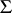A ring on which a an action ( "multiplication" ) of elements of the ring by elements from a fixed setis defined (an external law of composition), such that the following axioms are satisfied: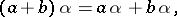(1)(2)

whereis an element ofwhile,,,are elements of the ring. In this way, the operators act as endomorphisms of the additive group, commuting with multiplication by an element of the ring. A ring with domain of operators, or, more succinctly, a-operator ring, can also be treated as a universal algebra with two binary operations (addition and multiplication) and with a setof unary operations linked by the usual ring identities as well as by the identities (1) and (2). The concepts of a-permissible subring, a-permissible ideal, a-operator isomorphism, and a-operator homomorphism can be defined in the same way as for groups with operators (cf. Operator group). If a-operator ringpossesses a unit element, then all ideals and all one-sided ideals of the ringare-permissible.

A ringis called a ring with a ring of operatorsif it is a-operator ring whose domain of operatorsis itself an associative ring, while for any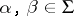and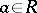the following equalities hold: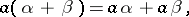(3)(4)

A ring with a ring of operators can also be defined as a ring which is simultaneously a-module and which satisfies axiom (2). Every ring can naturally be considered as an operator ring over the ring of integers.

For allfromandfrom, the elementis an annihilator of. Therefore, ifis a ring with operators without annihilators, then its ring of operatorsmust be commutative.

The most commonly studied rings with operators are those with an associative-commutative ring of operators possessing a unit element. This type of ring is usually called an algebra over a commutative ring, and also a linear algebra. The most commonly studied linear algebras are those over fields; the theory of these algebras is evolving in parallel with the general theory of rings (without operators).

How to Cite This Entry:
Ring with operators. Encyclopedia of Mathematics. URL: http://encyclopediaofmath.org/index.php?title=Ring_with_operators&oldid=17809
This article was adapted from an original article by K.A. Zhevlakov (originator), which appeared in Encyclopedia of Mathematics - ISBN 1402006098. See original article